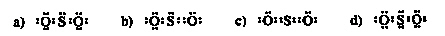Name:    Practice Test 2B

Multiple Choice
Identify the choice that best completes the statement or answers the question.

1.

An atom containing 19 protons, 20 neutrons and 19 electrons has a mass number of
 A) 39 B) 20 C) 38 D) 19

2.

[Kr] 5s2 4d10 is the electron configuration for
 A) As B) I C) Br D) Cd

3.

Which of the following is NOT correct?
 A) The proton has a charge of +1 B) The neutron has a charge of +1 C) The proton has a relative mass of 1 amu D) The electrons has a relative mass of about 1/1800 amu

4.

What is the correct formula for zinc acetate?
 A) Zn2C2H3O2 B) ZnC2H3O2 C) Zn(C2H3O2)4 D) Zn(C2H3O2)2

5.

Which of the following is the correct Lewis structure for SO2?A) a B) b C) c D) d

6.

Which of the following molecules is polar?
 A) HBr B) CF4 C) H2 D) CO2

7.

Which of the following combinations is INCORRECT?
 A) CuO, copper (II) oxide B) FeO, iron (II) oxide C) CCl4, carbon (IV) chloride D) PbCl4, lead (IV) chloride

8.

A particular ion has a formula 90Sr2+.  The number of electrons found in one of these ions is
 A) 36 B) 38 C) 2 D) 90

9.

Each atom of a specific element has the same
 A) atomic mass B) nuclear mass C) number of protons D) number of neutrons

10.

The correct formula for a compound between barium and oxygen would be
 A) Ba2O B) Ba2O2 C) BaO2 D) BaO

11.

Two isotopes of an element have masses and abundances as follows: 54.00 amu (20.0%) and 56.00 (80.00%).  What is the atomic mass of the element?
 A) 55 B) 54.2 C) 55.6 D) 54.4

12.

The correct formula for iron(III) nitride is
 A) Fe3NO3 B) Fe(NO3)3 C) Fe3N D) FeN

13.

Element X combines with phosphate to form X3(PO4)2.  Element X is
 A) Ca2+ B) Na+ C) N3– D) Al3+

14.

The concept that most of an atom’s mass is concentrated in a small nucleus surrounded by electrons was the contibution of
 A) Thomson B) Rutherford C) Dalton D) Chadwick

Completion
Complete each statement.

15.

The correct name for H3PO4 is ___________________

16.

The correct formula for barium hydroxide is ______________

17.

The correct name for MgCO3 is ________________